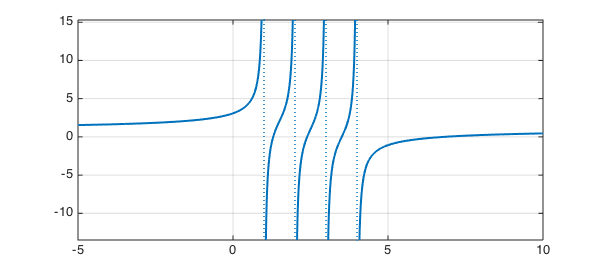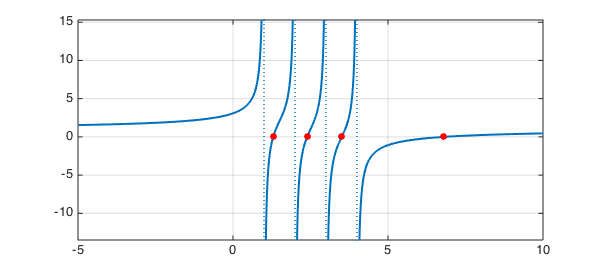Sometimes one needs to find the roots of a rational function expressed in partial fraction form, like this:

$$r(x) = 1 + \sum_{j=0}^N \frac{a_j}{b_j-x} .$$

For example, a "secular equation" of this kind arises in numerical linear algebra as part of the divide and conquer algorithm for computing eigenvalues of symmetric matrices (see  and p. 231 of ). If the coefficients $a_j$ are positive and the poles $b_j$ are distinct, then $r$ must switch from $+\infty$ to $-\infty$ as $x$ passes through each pole, and it follows that $r$ has exactly $N-1$ real zeros lying between the poles and also one more real zero lying to the right of all the poles.

Here is an example with $N=4$:

x = chebfun('x',[-5 10]);
for j = 1:4
f = 1 + 1./(1-x) + 1./(2-x) + 1./(3-x) + 1./(4-x);
end
hold off, plot(f,'linewidth',2), grid onChebfun can compute the roots:

format long, format compact
r = roots(f)
r =
1.000000000000000
1.296089645312119
2.000000000000000
2.392275290272984
3.000000000000000
3.507748705363648
4.000000000000000
6.803886359051248


Notice that the result is 8 numbers, including the poles as well as the roots. This is because Chebfun's convention is to regard a function as having a root at any point where it crosses between positive and negative values. If we don't want roots of that kind, we can execute instead

r = roots(f,'nojump')
r =
1.296089645312119
2.392275290272984
3.507748705363648
6.803886359051248


Let us add the roots to the plot.

hold on, plot(r,f(r),'.r','markersize',24)1. J. J. M. Cuppen, A divide and conquer method for the symmetric tridiagonal eigenproblem, Numerische Mathematik 36 (1980/81), 177-195.

2. L. N. Trefethen and D. Bau, III, Numerical Linear Algebra, SIAM, 1997.